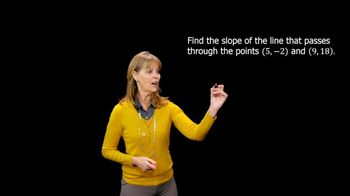Start typing, then use the up and down arrows to select an option from the list.
3:20 minutes
Problem 44
Textbook Question

# In Exercises 41–44, use the given conditions to write an equation for each line in point-slope form and slope-intercept form. Passing through (-3, 6) and perpendicular to the line whose equation is y = (1/3)x + 4Verified Solution
This video solution was recommended by our tutors as helpful for the problem above.
70views